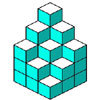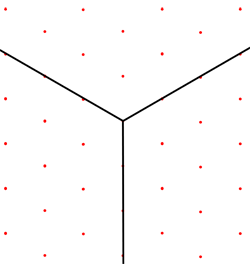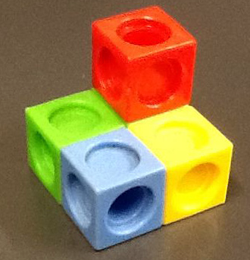#### You may also like### Soma - So Good

Can you mentally fit the 7 SOMA pieces together to make a cube? Can you do it in more than one way?### Nine Colours

Can you use small coloured cubes to make a 3 by 3 by 3 cube so that each face of the bigger cube contains one of each colour?### Take Ten

Is it possible to remove ten unit cubes from a 3 by 3 by 3 cube so that the surface area of the remaining solid is the same as the surface area of the original?

# Isometric Drawing

##### Age 11 to 14Challenge Level

If you are not familiar with Isometric Perspective, start by reading our article 3D Drawing.The diagram on the right shows a set of three coordinate axes on an isometric drawing.  What is the angle between each pair of axes?

Because the angles between the three axes are all the same, each one must be 120 degrees.

Is it possible to keep the distance between all adjacent grid points equal, and have some other angles between the axes?

The photo below shows a multilink structure.  Draw it on isometric paper.

Which properties of the original structure does your drawing preserve, which are not preserved?  You should think about:

• the relationship between the lengths of the edges of the cubes
• the angles between them
• parallel and perpendicular linesHow does your drawing compare with a drawing of the same view of this structure in Oblique Projection?  What are the advantages of each, what are the disadvantages?